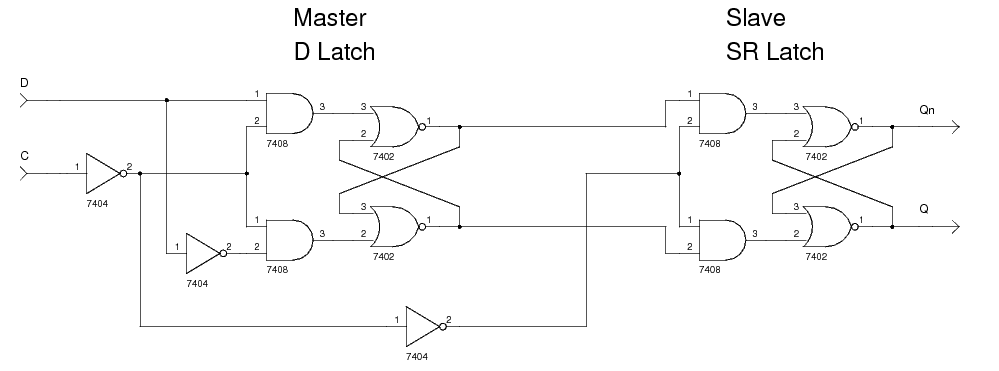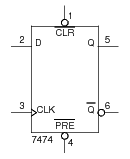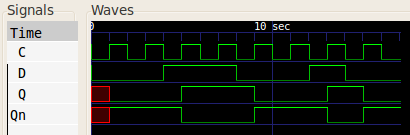# D Flip-Flop (edge-triggered)

A D flip-flop is used in clocked sequential logic circuits to store one bit of data.

The D flip-flop described here is positive edge-triggered which means that the input which is stored is that input which is seen when the input clock transitions from `0` to `1`. This flip-flop is built from two gated latches: one a master D latch, and the other a slave SR latch. The master takes the flip-flops inputs: `D` (data) and `C` (clock). The clock input is inverted and fed to the D latch's gate input. The slave takes the master's outputs as inputs (`Q` to `S` and `Qn` to `R`), and the complement of the master's clock input. The slave's outputs are the flip-flop's outputs. This difference in clock inputs between the two latches disconnects them and eliminates the transparency between the flip-flop's inputs and outputs.

The schematic below shows a positive edge-triggered D flip-flop. The input `D` is used to set and reset the flip-flop's data. The clock input `C` is used to control both the master and slave latches making sure only one of the latches can set its data at any given time. When `C` has the value `1`, the slave can set its data and the master cannot. When `C` has the value `0`, the master can set its data and the slave cannot. When `C` transitions from `0` to `1` the master has its outputs set which reflect the flip-flop's inputs when the transition occurred. The outputs `Q` and `Qn` are the flip-flop's stored data and the complement of the flip-flop's stored data respectively.The schematic symbol for a 7474 edge-triggered D flip-flop is shown below. This chip has inputs to asynchronously clear and set the flip-flop's data.## Example

The following function table shows the operation of a D flip-flop. The column header `Q(t+1)` means "the value of `Q` at the start of the next clock period", similarly for `Qn(t+1)`.

`D` `Q(t+1)` `Qn(t+1)` Meaning
`0``0``1`Reset
`1``1``0`Set

## Verilog

Below is the Verilog code for a structural model of a positive edge-triggered D flip-flop. The code for the gated D and SR latches is also shown for completeness.

``` module d_flip_flop_edge_triggered(Q, Qn, C, D); output Q; output Qn; input C; input D; wire Cn; // Control input to the D latch. wire Cnn; // Control input to the SR latch. wire DQ; // Output from the D latch, input to the gated SR latch. wire DQn; // Output from the D latch, input to the gated SR latch. not(Cn, C); not(Cnn, Cn); d_latch dl(DQ, DQn, Cn, D); sr_latch_gated sr(Q, Qn, Cnn, DQ, DQn); endmodule // d_flip_flop_edge_triggered module d_latch(Q, Qn, G, D); output Q; output Qn; input G; input D; wire Dn; wire D1; wire Dn1; not(Dn, D); and(D1, G, D); and(Dn1, G, Dn); nor(Qn, D1, Q); nor(Q, Dn1, Qn); endmodule // d_latch module sr_latch_gated(Q, Qn, G, S, R); output Q; output Qn; input G; input S; input R; wire S1; wire R1; and(S1, G, S); and(R1, G, R); nor(Qn, S1, Q); nor(Q, R1, Qn); endmodule // sr_latch_gated ```

A simulation with test inputs gave the following wave form:## References

Kleitz, W. Digital Microprocessor Fundamentals. 3rd Edition. Prentice Hall, 2000.
Mano, M. Morris, and Kime, Charles R. Logic and Computer Design Fundamentals. 2nd Edition. Prentice Hall, 2000.